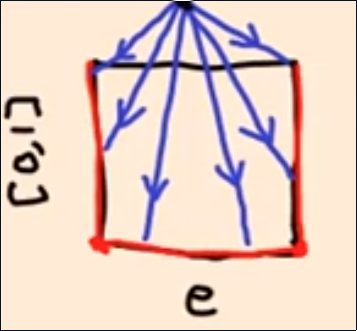## § CW Complexes and HEP

If $X$ is a CW complex and $A$ is a closed subcomplex, then it has the HEP. A closed subcomplex is a union of closed cells of $X$ such that $X$ is obtained by adding more cells to $A$.

#### § Lemma

If $e$ is a disk, then there is a continuous map from $e \times [0, 1]$ to $\partial e \times [0, 1] \cup (e \times \{ 0 \})$.#### § Lemma

If $X$ is obtained from $A$ by attaching one $k$-cell, then $(X, A)$ has HEP. Given a homotopy $h_t: A \times [0, 1] \rightarrow Y$ and a new homotopy $F_0: X \rightarrow Y$ such that $F_0|A = h_t$, we want to complete $F$ such that $F_t|A = h_t$. The only part I don't know where to define $F$ on is the new added $e$ portion. So I need to construct $H$ on $e \times [0, 1]$. Use the previous map to get to $e \times [0, 1] \cup (e \times \{0\})$. This is in the domain of $F_0$ or $h_t$, and thus we are done.

#### § CW Complexes have HEP

Induction on lemma. base case is empty set.

#### § Connected 1D CW Complex

Theorem: any connected 1D CW complex is homotopic to wedge of circles.
• Find a contractible subcomplex $A$ of $X$ that passes through all $0$ cells.
• By HEP, $X \simeq X/A$. $X/A$ has only one zero-cell and other one cells. One cells are only attached to zero cells. Hence, is a wedge of circles.
• The idea to find a contractible subcomplex is to put a partial order on the set of all contractible cell complexes by inclusion.
• Pick a maximal element with respect to this partial order.
• Claim: maximal element must contain all zero cells. Suppose not. Then I can add the new zero cell into the maximal element (why does it remain contractible? Fishy!)